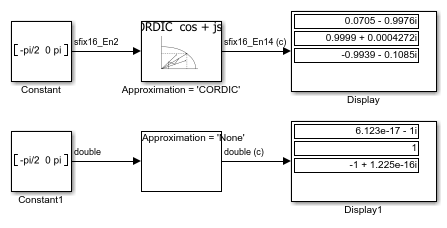Main Content

# Trigonometric Function Block Behavior for Complex Exponential Output

This example compares the complex exponential output for two different configurations of the Trigonometric Function block.When the Approximation method is `CORDIC`, the input data type can be fixed point, in this case: `fixdt(1,16,2)`. The output data type is `fixdt(1,16,14)` because the output fraction length equals the input word length minus two.

When the Approximation method is `None`, the input data type must be floating point. The output data type is the same as the input data type.

## Support

#### Model-Based Design for Embedded Control Systems

Download white paper